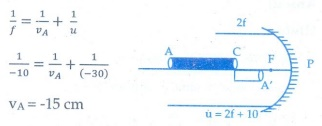# UNIT VI: Ray Optics - Online Test

Q1. The speed of light in an isotropic medium depends on,
Explaination / Solution:

c = vλ, v is independent of medium

Q2. A rod of length 10 cm lies along the principal axis of a concave mirror of focal length 10 cm in such a way that its end closer to the pole is 20 cm away from the mirror. The length of the image is,
Explaination / Solution:

End A of the rod acts as an object for mirror and A' will be its image. By mirror formula, image distance of A is VA.VA = -15cm

Also image distance of C

Vc= -20 cm

The length of image = | VA-VC |

= | -15-(20) | = 5cm

Q3. An object is placed in front of a convex mirror of focal length of f and the maximum and minimum distance of an object from the mirror such that the image formed is real and magnified.
Explaination / Solution:

Since the nature of image produced by a convex mirror is always virtual, erect and diminished.

Q4. For light incident from air on a slab of refractive index 2, the maximum possible angle of refraction is,
Explaination / Solution:

n= 2;

i=90◦

n = sin i / sin r

sin r = sin i / n

= sin 90◦/2 = ½

r = sin-1(1/2)  = 30◦

Q5. If the velocity and wavelength of light in air is Va and λa and that in water is Vw and λ , then the refractive index of water is,
Explaination / Solution:

n = speed of light in vacuum / speed of light in medium =Va / Vw

Q6. Stars twinkle due to,
Explaination / Solution:
No Explaination.

Q7. When a biconvex lens of glass having refractive index 1.47 is dipped in a liquid, it acts as a plane sheet of glass. This implies that the liquid must have refractive index,
Explaination / Solution:

Since the liquid act as a plane sheet of glass its refractive index is equal to that of glass.

Q8. The radius of curvature of curved surface at a thin planoconvex lens is 10 cm and the refractive index is 1.5. If the plane surface is silvered, then the focal length will be,
Explaination / Solution:

For a plano-convex lens with its plane surface silvered

1/f = 2(n − 1)/R

f  = R / 2(n − 1) = 10 / 2(1.5 − 1) = 10 / 2(0.5) = 10 cm

Q9. An air bubble in glass slab of refractive index 1.5 (near normal incidence) is 5 cm deep when viewed from one surface and 3 cm deep when viewed from the opposite face. The thickness of the slab is,
Explaination / Solution:

n - 1.5 ; d' = nd

In one side bubble depth is 5 cm

Original depth = 1.5 x 5 = 7.5 cm

On the other side depth is 3 cm

Original depth = 1.5 x 3 = 4.5 cm

Thickness is 7.5 + 4.5 = 12 cm

Q10. A ray of light travelling in a transparent medium of refractive index n falls, on a surface separating the medium from air at an angle of incidents of 45º. The ray can undergo total internal reflection for the following n,
Explaination / Solution:

For total internal reflection

i > C;

sin i > sin C;

sin 45◦ > 1/n

1/√2 > 1/n

n>√2

n>1.414

Here, n=1.5